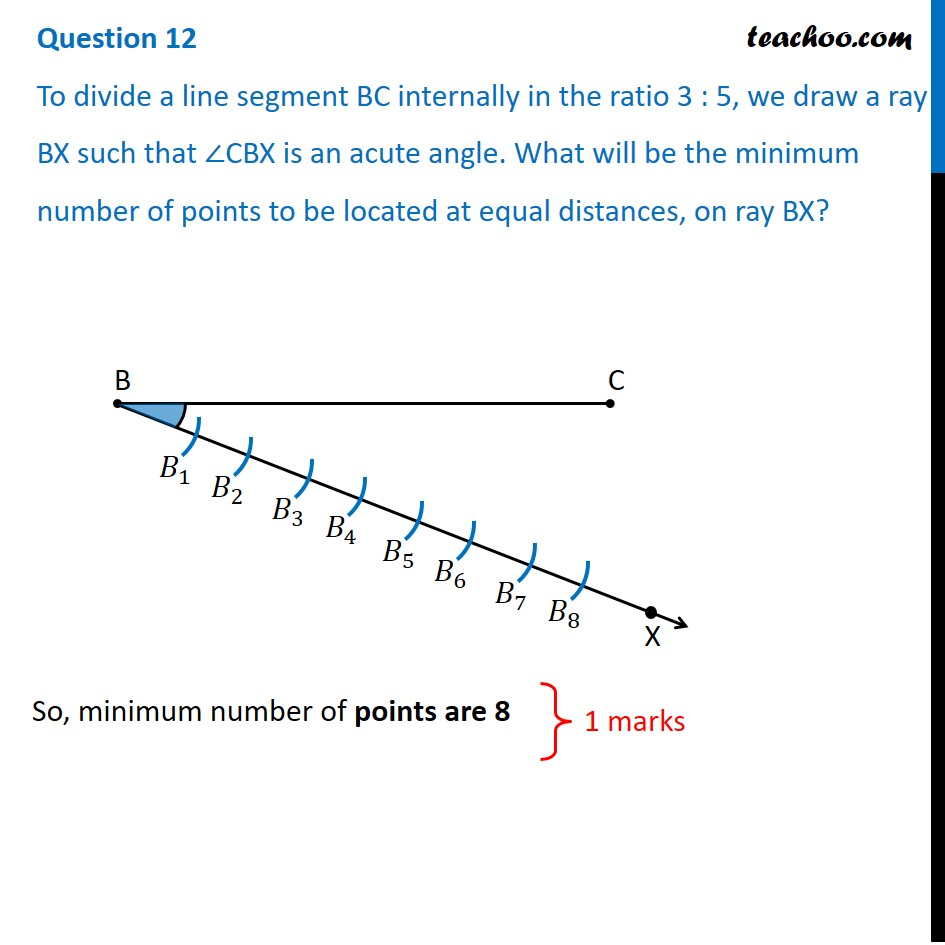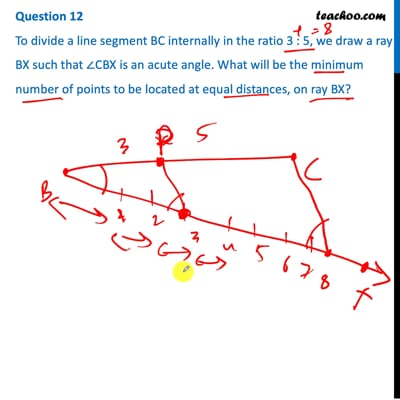CBSE Class 10 Sample Paper for 2021 Boards - Maths Basic

Class 10
Solutions of Sample Papers for Class 10 Boards

## To divide a line segment BC internally in the ratio 3 : 5, we draw a ray BX such that ∠CBX is an acute angle. What will be the minimum number of points to be located at equal distances, on ray BX?This video is only available for Teachoo black users

### Transcript

Question 12 To divide a line segment BC internally in the ratio 3 : 5, we draw a ray BX such that ∠CBX is an acute angle. What will be the minimum number of points to be located at equal distances, on ray BX? So, minimum number of points are 8 So, minimum number of points are 8 1 marks